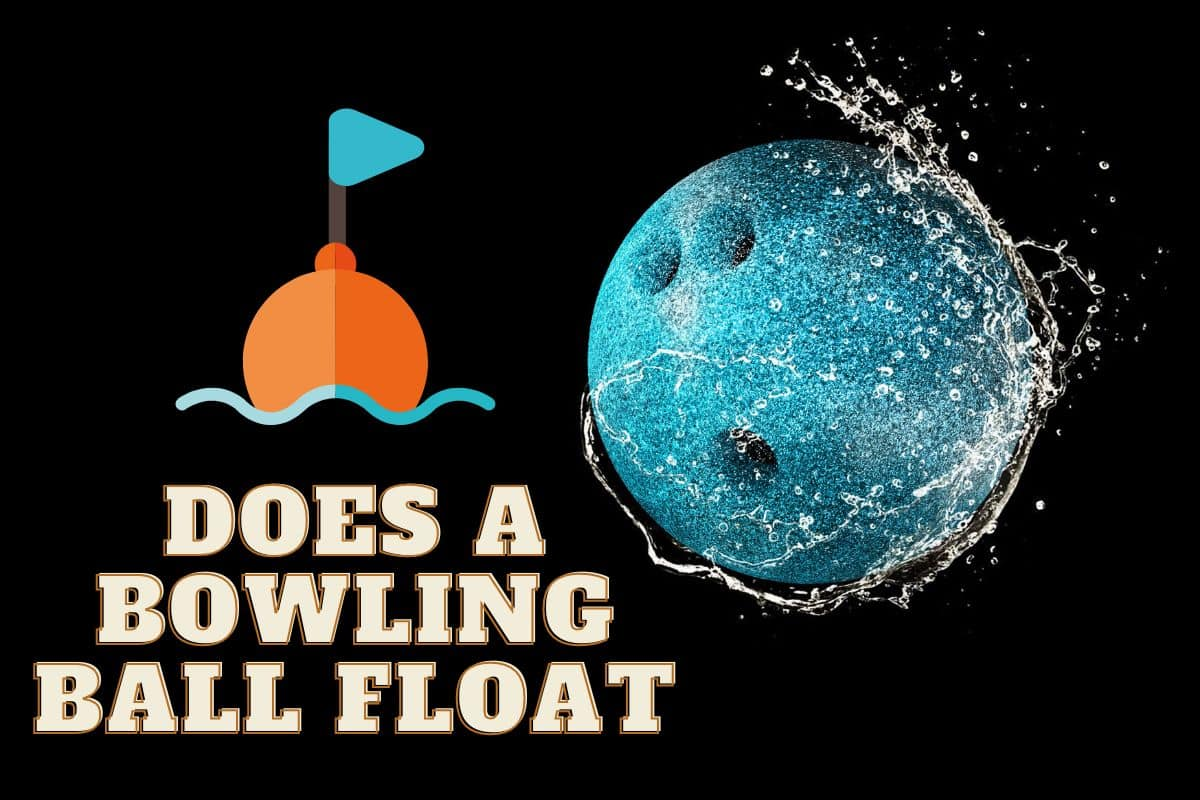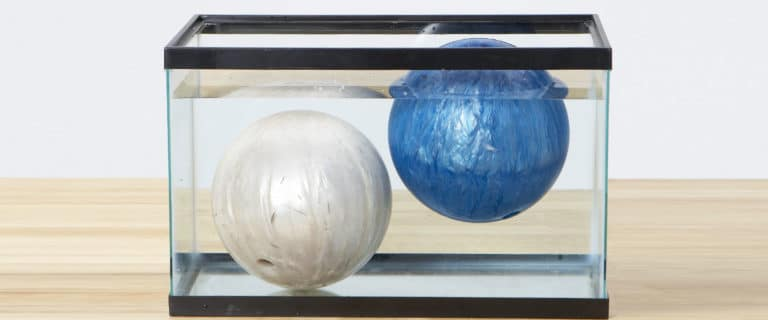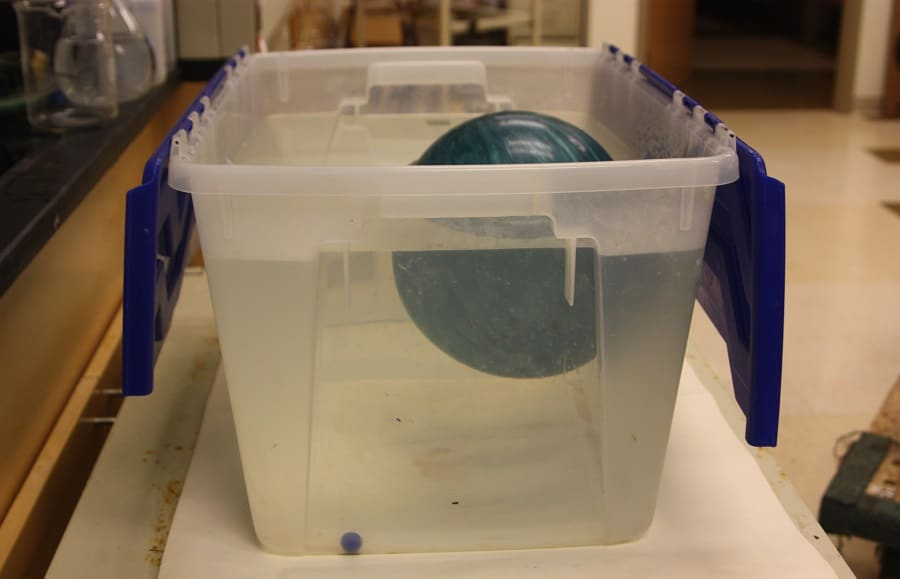# Does a Bowling Ball Float or Sink?

Bowling balls come in a wide variety of sorts, and depending on the type, they may or may not float. The composition and weight of a ball are the primary determinants of whether it floats or not. If you've ever wondered if a bowling ball will float, this post is for you.

If you have been bowling for a while, you may have asked yourself if a bowling ball can float. This is quite a puzzling question, as bowling balls are made of solid, heavy material and seem like they would sink into the water. So, does a bowling ball float? The answer is not a simple yes or no. The ball's mass and the density of the water itself are the primary variables at play here. Typically, if a ball weighs more than 12 pounds, it will sink. However, if the ball is lighter and the water is denser, it may be possible for the ball to float. If you are wondering if your bowling ball will float in a pool, this post will provide some insight into how bowling balls behave in different types of water. If this sounds interesting to you, read on!

## Why Some Bowling Balls Float While Others Sink

To understand why some bowling balls float, it is important to understand the concept of density. Density is the main factor determining whether an object will float or sink. If the ball is denser than the water it is placed in, it will sink, and if it is less dense than the water, it will float. The density of the ball is affected by two factors: volume and weight of the ball. Another main factor affecting the density of the ball and whether or not the ball will sink or float is the material used to make the ball. Bowling balls are typically made of three materials: Plastic, Urethane, and Reactive resin.

• Plastic: Plastic bowling balls are the lightest and least dense, so they tend to float. Just like many other household plastic items, these balls are made of a lightweight material that is not dense enough to sink.
• Urethane: Urethane is a medium-weight material that is denser than plastic. Urethane is also resistant to water and can be used as a sealant. Most urethane bowling balls will float in water, but denser variations may sink.
• Reactive resin: Bowling balls made of reactive resin are heavier than plastic and urethane balls. They are, therefore, more probable to sink than the other two types of bowling balls.

It is important to note that the density of water is about 1g/cm3, and that of a ball starts at about 0.67g/cm3. This means that most bowling balls will float in water. Note that if your bowling ball's density is more than 1g/cm3, it will sink.

## Do Bowling Balls Float or Sink in Fresh and Salty Water?If we learned anything from high school physics, it's that items will float if their density is lower than the liquid they are placed in. The identical thing will typically float in freshwater and sink in saltwater because saltwater is denser than freshwater. The standard bowling ball will float in freshwater, so it stands to reason that it will also float in saline water. Therefore, on salty water, the majority of bowling balls will float.

Generally speaking, a bowling ball will float in the ocean due to the high density of the ocean water. You, therefore, don't have to worry about your bowling ball sinking to the bottom of the ocean. If you drop it, it will float, and you can easily retrieve it.

## Will A Bowling Ball Sink or Float In a Swimming Pool?

A swimming pool typically has additional chemicals, such as chlorine. Swimming pools also tend to have a higher salt composition than fresh water. You might think that a bowling ball would sink in the water due to its weight; however, the chemical composition of the pool water makes it denser than the bowling ball. As a result, if the bowling ball is dense, it will most definitely float in a swimming pool.

## How to measure the density of a bowling ballIn this quick experiment, we will do two things:

1. Measure the weight of a bowling ball
2. Measure the density of a bowling ball

### a) How to measure the weight of a bowling ball

To measure the weight of a bowling ball, all you need to do is make use of a digital weighing balance to measure and record the weight. Take note that according to Professional Bowlers Association (PBA), the weight of standard bowling balls ranges from 8 to 16 pounds.

### b) How to measure the density of a bowling ball

Wrap a piece of string around the widest part of a bowling ball to measure the circumference of the ball. Take note that according to regulations, the circumference of a bowling ball should be in the range of 26.704 – 27.002 inches. Essentially, that means that all regulation bowling balls are of the same size. That being said, let’s proceed…

• Assuming you have measured the circumference of the bowling ball and found it to be 27 inches, convert that into centimeters using the conversion factor of 1 inch = 2.54 cm
• Do the math: 27 inches x 2.54cm = 68.58cm
• To find the volume of the bowling ball sphere, we first need to find the radius. All that derives from this formula:

The volume of a sphere = 4/3 x  x r3 where r is the radius of the ball and

Circumference = 2

Meaning r = 68.58cm/ 2 = 10.92cm

Volume = 4/3 x  x r3

= 4/3 x 3.14 x 10.923 cm

= 5452 cm3

To find the density of the ball, use the formula:

Density = Mass/ Volume

• Assume the mass of the ball is = 8 pounds

Pro Tip! Change the weight to grams using the conversion factor: 1 pound = 453.6 grams

Therefore: Density = (8 x 453.6) grams x Volume

= 3632g/ 5452cm3

= 0.67g/cm3

Remember that water has a density of 1.0g/cm3. Anything that has a density of less than that of water, floats on it. Similarly, anything with a density of more than that of water sinks in it. Now you know why an 8-pound bowling ball with a density of 0.67g/cm3 floats in water while a 13-pound bowling ball with a density of 1.08g/cm3 sinks.

## Bottom Line

We've established that generally speaking, bowling balls will float if dropped into a pool of water. The reason for this is the low density of bowling balls compared to water. The ball will always maintain its buoyancy when placed in salt water, as found in a swimming pool or the ocean. Even though it might seem like all balls float, there are some heavy balls out there than can weigh up to 16 pounds. Such balls may not float in water due to their higher density. When purchasing a bowling ball, you can always test it by submerging it in a full bucket of water to check if it floats or sinks. By doing this, you can determine the buoyancy of the ball before making a purchase.